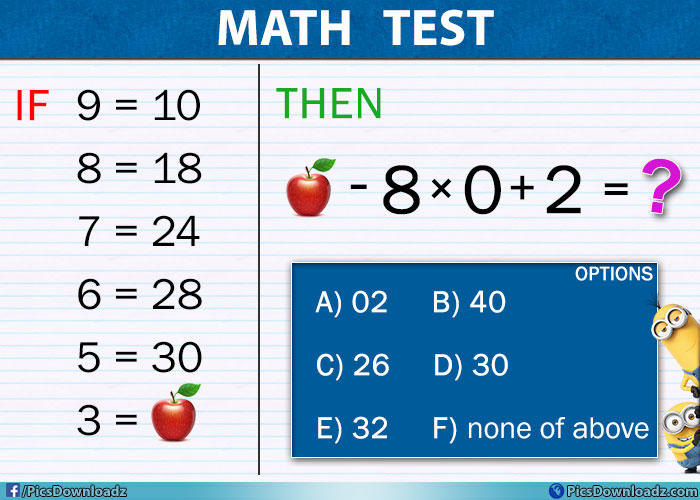This post may contain affiliate links. This means if you click on the link and purchase the item, We will receive an affiliate commission at no extra cost to you. See Our Affiliate Policy for more info.

If 9=10 & 3=Apple, Then Apple-8×0+2 = ?? Solve this difficult maths puzzle problem

1
4182

Solve this genius maths puzzle problem! 95% of people will fail to find the answer to this brain teaser maths puzzle!

Getting expert day by day? Here is our another genius maths puzzle problem for you. Solving puzzles is all about fun and knowing some logical tricks which help’s us in various exams and interview.

Well, this puzzle is divided into two parts. First, you have to figure out the logic behind these numbers and how they relate to each other and then find out which number replace the Apple.

Now in the second half, put the apple value in the equation and find the answer. Best of luck!

Puzzle Question:

1. Find the value of Apple

9 = 10

8 = 18

7 = 24

6 = 28

5 = 30

3 = Apple

2. Solve the equation

Apple – 8 × 0 + 2 = ??

Maths Puzzle Problem Image:Options:

A) 02,   B) 40,   C) 26,   D) 30

E) 32,   F) none of above

Did you find your answer in the options? Yes-No? Share your answer in the comment section below. Keep scrolling for the solution of this puzzle.

.

A

N

S

W

E

R

.

1. Find the value of Apple

Did you got Apple = 24? Sorry, this one is wrong, check the logic of the puzzle and correct value of apple below;

9 = 10           ⇒    9 × 1 + 1 = 10

8 = 18           ⇒    8 × 2 + 2 = 18

7 = 24           ⇒    7 × 3 + 3 = 24

6 = 28           ⇒    6 × 4 + 4 = 28

5 = 30           ⇒    5 × 5 + 5 = 30

4 =                 ⇒    4 × 6 + 6 = 30 (skipped in question)

3 = Apple     ⇒    3 × 7 + 7 = 28

Have different method to solve this part? Share with us via comments.

2. Solve the equation

⇒  Apple – 8 × 0 + 2 = ??   (Now Apple = 28)

⇒  28 – 8 × 0 + 2  (Do multiplication first i.e  -8 × 0 = 0)

⇒   28 + 2 = 30 Ans.

So, the correct option is D) 30 Ans.

Is your answer is Correct? Congratulation, you are awesome! Even if get wrong, don’t worry, you have just learned something new today. This is how these logical maths puzzles work.

Like | Share | Comment

Like us on facebook for more interesting puzzles questions and funny stories. If you love puzzle then you should definitely join our facebook group;

1.Aseem Mishra